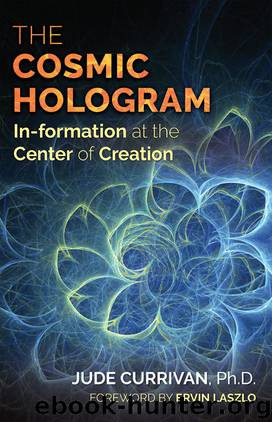# The Cosmic Hologram by Jude CurrivanAuthor:Jude Currivan
Language: eng
Format: epub
Tags: New Science
Publisher: Inner Traditions / Bear & Company
Published: 2017-02-02T16:00:00+00:00

HARMONIC ORDER

When I was at school many years ago, and well before the invention of pocket calculators, in my math class I learned how to use two indispensable tools: log tables and a slide rule. And while electronic calculators and computers have long since made both obsolete, how and why they worked is as pertinent—and with the understanding of the cosmic hologram and the harmonic nature of reality—even more relevant than ever. Let me explain why.

What they both enabled was for otherwise complicated sums such as multiplication, division, and calculating square roots of numbers to be dramatically simplified. They did this by using logarithms, which convert multiplication into addition and division into subtraction—much easier!

You’ll probably be grateful that I’m not about to go into the technicalities of logarithms. What’s key for us here though is to appreciate that they operate in the way they do because essentially they’re harmonic. This is owing to their being exponents (or powers) of a base number, such as 10, where the base is multiplied by itself however many times to arrive at a required number.

To explain this numerically, we need a bit of math. The log of any number x (say 100) is the power to which the base (in this case 10) would need to be raised to equal x; in this case 2, as 100 is 10 × 10. So the log of 100 in base 10 is 2, or log10(100) = 2.

This allows the conversion of numbers to their log equivalent, and vice versa, and the harmonic mathematical properties of logs to be then used to simplify complicated calculations. For optimum ease, base 10 (or common) logs are used in general science and engineering and base 2 (or binary) logs in computing science. Base e (or natural) logarithmic relationships, though, where e is Euler’s number, pervade mathematics and are especially important given the deep connection between e and the complex plane.

An essential consequence of their harmonic nature is that logarithms are rather like geometric fractals in that they are self-similar and scale up and down by factors of their numerical base. Logs are found through many universal relationships, and logarithmic spirals are key features of numerous evolutionary processes. We even see and hear the world logarithmically, because our eyes respond in this way to the brightness of visible light and our ears to the logarithmic decibel scale of the frequencies of audible sounds. Such logarithmic sensory perception enables us to see and hear across a wider range of visual and acoustic stimuli without overwhelming our senses.

Natural logarithms pervade Fourier transforms that are basic to the holographic principle. Most significant of all perhaps, they anchor the fundamental equation for informational entropy and so again reveal the nature of reality in innately harmonic, fractal, and holographic terms.

A while back we explored how nothing is ultimately random and considered a common distribution of data, such as the heights of a group of people, that overall fit into a so-called Gaussian distribution curve in the shape of a bell and where the average measure of the group correlates to the peak of the bell.